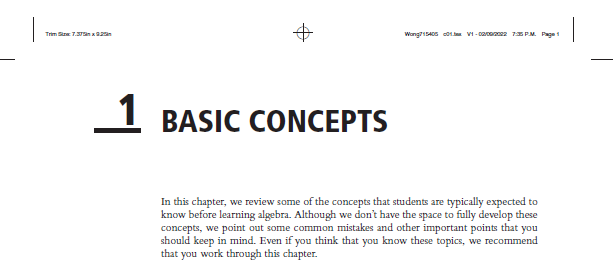# Errata in Practical AlgebraHere is a list of errata in Practical Algebra: A Self-Teaching Guide (3rd edition) by Bobson Wong, Larisa Bukalov, and Steve Slavin. To report an error, fill out the form at http://bobsonwong.com/contact.

p. 193
Exercise #11 should read slope of 3/7, not slope of -3/7.

p. 246 (noted by Nicole Myers)
Example 8.16 should read Calculate (9x3 − 49x + 40) ÷ (3x + 8), not Calculate (9x3 − 49x + 40) ÷ (3x + 4).

p. 401
In Figure 12.7, the horizontal change in the non-linear function on the right next to m = 5/2 should be labeled “2,” not “1” (see corrected figure below):